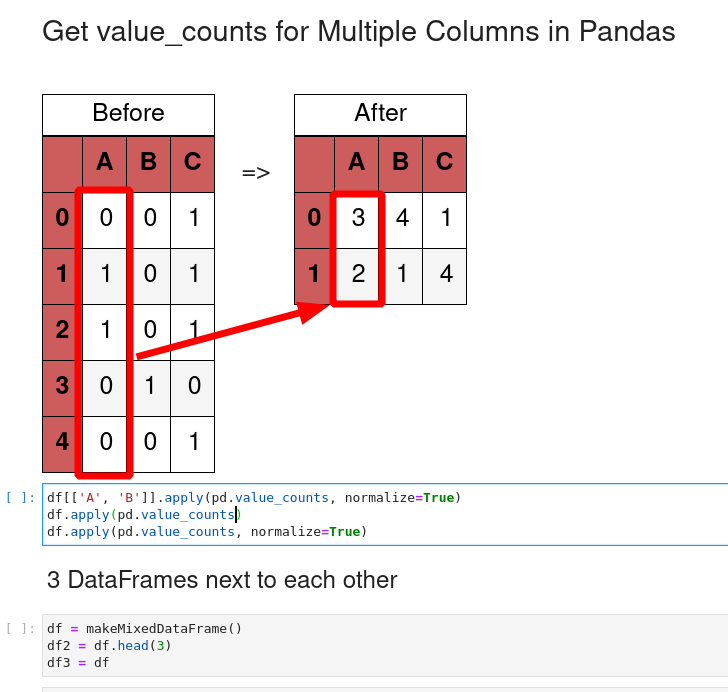Need to get value_counts for multiple columns in Pandas DataFrame? In this article we will cover several options to get value counts for multiple columns or the whole DatFrame.

## Setup

Let's create a sample DataFrame which will be used in the next examples:

``````import numpy as np
import pandas as pd

df = pd.DataFrame(np.random.randint(0, 2, (5, 3)), columns=list('ABC'))
``````

The tabular representation of the DataFrame is:

A B C
0 1 0 1
1 0 1 1
2 0 1 1
3 0 0 1
4 0 1 1

## Step 1: Apply value_counts on several columns

Let's start with applying the function `value_counts()` on several columns. This can be done by using the function `apply()`.

We can list the columns of our interest:

``````df[['A', 'B']].apply(pd.value_counts)
``````

The results is a DataFrame with the count for columns:

A B
0 4 2
1 1 3

## Step 2: Apply value_counts with parameters

What if we like to use `value_counts()` on multiple columns with parameters? Then we can pass them the the `apply()` function as:

``````df[['A', 'B']].apply(pd.value_counts, normalize=True)
``````

The result is the normalized count of columns A and B:

A B
0 0.8 0.4
1 0.2 0.6## Step 3: Apply value_counts on all columns

To apply `value_counts()` on every column in a DataFrame we can use the same syntax as before:

``````df.apply(pd.value_counts)
``````

The result is the count of each column:

A B C
0 4 2 NaN
1 1 3 5.0

## Step 4: Simulate value_counts with melt

Finally let's check how we can use advanced analytics in order to manipulate data.

We will use the `melt()` function in order to reshape the original DataFrame and get count for columns.

The first step is to use `melt()`:

``````df.melt(var_name='column', value_name='value')
``````

This will change data into two columns - in other words - DataFrame will be represented row wise in columns:

• column - the source column
• value - the value of the column
column value
0 A 1
1 A 0
2 A 0
3 A 0
4 A 0

Now we can apply `value_counts()`:

``````df.melt(var_name='column', value_name='value').value_counts()
``````

To get result as:

``````column  value
C       1        5
A       0        4
B       1        3
0        2
A       1        1
dtype: int64
``````

or we can display the results as a DataFrame with sorted counts:

``````(pd.DataFrame(
df.melt(var_name='column', value_name='value').value_counts())
.sort_values(by=['column']).rename(columns={0: 'count'}))
``````
count
column value
A 0 4
1 1
B 1 3
0 2
C 1 5
× Pro Tip 1
Advanced users can go further and combine:
`pd.crosstab()`
and
`df.melt`
``````pd.crosstab(**df.melt(var_name='columns', value_name='index'))
``````

This will result into:

columns A B C
index
0 4 2 0
1 1 3 5

## Conclusion

To summarize we saw how to apply `value_counts()` on multiple columns. We covered how to use `value_counts()` with parameters and for every column in DataFrame.

Finally we discussed advanced data analytics techniques to get count of value for multiple columns.